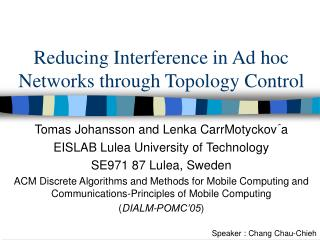Download PresentationReducing Interference in Ad hoc Networks through Topology ControlReducing Interference in Ad hoc Networks through Topology Control - PowerPoint PPT Presentation

Download PresentationReducing Interference in Ad hoc Networks through Topology Control
An Image/Link below is provided (as is) to download presentation

Download Policy: Content on the Website is provided to you AS IS for your information and personal use and may not be sold / licensed / shared on other websites without getting consent from its author. While downloading, if for some reason you are not able to download a presentation, the publisher may have deleted the file from their server.

- - - - - - - - - - - - - - - - - - - - - - - - - - - E N D - - - - - - - - - - - - - - - - - - - - - - - - - - -
Presentation Transcript

1. Reducing Interference in Ad hoc Networks through Topology Control Tomas Johansson and Lenka CarrMotyckov´a EISLAB Lulea University of Technology SE971 87 Lulea, Sweden ACM Discrete Algorithms and Methods for Mobile Computing and Communications-Principles of Mobile Computing (DIALM-POMC’05) Speaker : Chang Chau-Chieh

2. Outline • Introduction • Interference metrics • Average Path Interference algorithm (API) • Related works • Simulation • Conclusion

3. Introduction • Topology Control • Increase the network lifetime • Minimize the interference • Using interference model • Evaluate the topology

4. Introduction • G=(V,E) : Euclidian graph • V : network nodes • E : communication links

5. Introduction • GTC= (V,ETC) : t-spanner graph • the shortest path in GTCbetween any two nodes is at most t times longer than the shortest path between these nodes in G

6. Interference metrics – (1) • Interference model • the maximum coverage for any node

7. Interference metrics – (2) • The metrics do not consider • the paths in the graph • the interference in general

8. Interference metrics – (2) (3) • Interference-optimal path (IoptPuv) • Interference-optimal path with the shortest path (SPuv)

9. Interference metrics – (4) • Maximum interference difference metric

10. Average Path Interference algorithm (API) • Computing a Gabriel graph • Reducing the graph

11. API- Step 1

12. API- Step 2

13. API

14. Related works • Low Interference Spanner Establisher (LISE) • XTC algorithm

15. LISE • GTC= (V,ETC) : t-spanner graph • the shortest path in GTCbetween any two nodes is at most t times longer than the shortest path between these nodes in G

16. e2 LISE • Interference model Cov(e1) = 5 Cov(e2) = 1 e1 S

17. LISE

18. XTC • Nodes needs local knowledge. • Degree : 6 • GXTC does not contain any cycles of length 3

19. XTC

20. Simulation

21. Simulation Results

22. Simulation Results

23. Simulation Results

24. Simulation Results

25. Conclusion • We also propose a new topology control algorithm • Produce an energy-spanning graph • Established a new interference metrics • Low interference graph

26. Thank You.